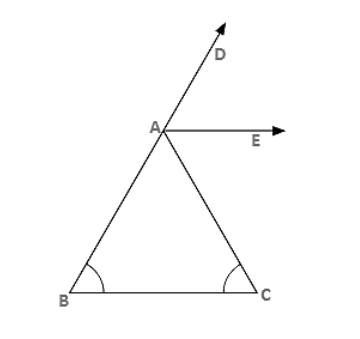# In figure 9.38, AE bisects ∠CAD and ∠B = ∠C. Prove that AE ∥ BC.`
Question:

In figure 9.38, AE bisects ∠CAD and ∠B = ∠C. Prove that AE ∥ BC.Solution:

Let ∠B = ∠C = x

Then,

∠CAD = ∠B + ∠C = 2x (exterior angle)